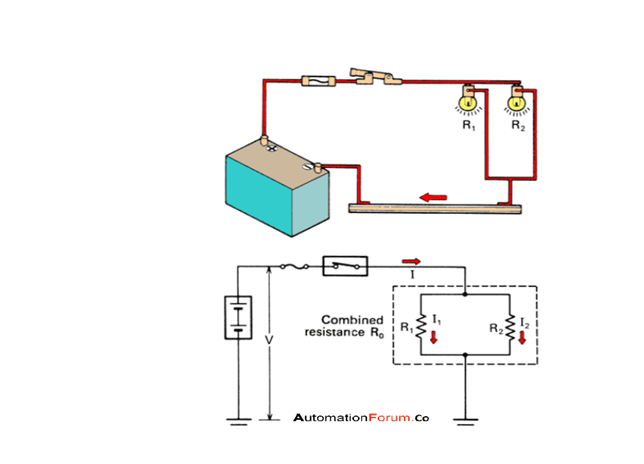# what are the Series and parallel circuit? which one is better parallel or series circuit## Series circuit

A series circuit is a simple circuit in which the components are connected in tandem. The conductors, protection devices loads, and power sources are connected with only one path to ground for current flow. All loads of the circuit carries same and equal current and the total resistance will be the sum of resistance around the circuit. The resistance of each device will be different and the amount of current flow will be the same for each device. But the voltage across the device would be different

If the path of the flow is broken then there won’t be any current flow an example for that would be a series LED light if one light goes off then the entire string won’t work

### Characteristics of series circuits

• It has only one path to ground so the electron must go through each component to get back to the ground
• All loads are placed in series so
• The entire circuit will be disabled by an open circuit
• The voltage will be shared between the loads
• The flow of current will be the same throughout the circuit
• The resistance of each load could be different
• The current value will always be the same at any point in a series circuit

## Parallel circuits

A parallel circuit has more than one path for the current flow, the same voltage is applied to each branch, if the load resistance in each branch is same then the current in each branch will be same, and it will be different for different load resistance.

In this circuit, the components and load are arranged in a manner that the current divides between them. If one branch is broken it won’t interrupt the current flow it will flow to the other branch

Each outlet has a wire which is connected to the current-carrying wire of the circuit and also a neutral wire or ground wire is connected to the return wire of the circuit. The sum of current flowing through each outlet is the total current flow through the circuit.

### Characteristics of the parallel circuit

• There is more than one pathway for the electron flow
• When the cells are connected in parallel the voltage will remain the same as the highest single cell
• If the components are connected in parallel the delivered voltage is not reduced
• They need more conducting wire
• Each item has its own pathway
• Resistance can be decreased by adding more pathway

### Which is better parallel or series circuit

• In series when the device is added overall resistance increases which decreases the current, but in parallel when devices are added overall resistance won’t increase
• Voltage is divided when connected in series and the load won’t get the proper voltage but there is no such problem in parallel connection
• If the pathway for one device breaks it won’t affect the other device in parallel but in series, it will affect the other device

so the parallel connection is better

Scroll to Top